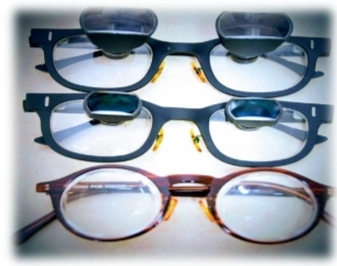## Optics of low vision devices

Low vision aids are mainly used this people who are unable to see and low vision devices are mainly used to magnify .All low vision devices are computed this formula , M=D/4, here M= magnification , D = dioptric power . This formula assumped that the unaided eye the patient can sustain just enough accommodation to hold the matter at 25 cm . For spectacles and magnifiers this formula can be written M=D+A/2.5 , where A is the amplitude of accommodation .
Magnification of low vision devices :- low vision devices make use of 4 type of magnifications . They are relative size, relative distance , angular and electro optical .
Relative size :- Relative size means enlargement of the size of the object ,it does not use an optical system. It is normally used to bigger the object
Relative distance magnification :-
Relative distance magnification achieved by moving the object of regard towards a person to subtend a larger image on the retina .
Angular magnification :-
Angular magnification achieved by the apparent change of size of the object compared with the true size of the object.
Electro – optical magnification :-
Electro optical magnification is produced by electronic systems which enlarge the objects by using computer .
Hand magnifiers optics :- Mainly optics of hand magnifiers are difference between front vertex power (fv) , back vertex power ( bv) and equivalent power of the hand magnifier (fm)
With a plano convex hand magnifier fc is closest to the front vertex power fv but back vertex power is greater
In a bi-convex lens of equal surface powers , front vertex power and back vertex power are equal but power of the hand magnifier is slightly less because the principle planes are inside the lens .
Fe = Fm+Fa-z.FmFa
Where Fe is the equivalent power of the system , Fm is the equivalent power of the hand magnifiers , Fa is the power of accommodation and Z is the eye to hand magnifiers distance ,
When Fe = Fm
If the eye magnifier separation is exactly the same as the focal length , z is equal to fm ,
Fe= Fm+Fa – z.FmFa,
Thus , Fe = Fm+Fa – fm.Fm Fa
fm .Fm =1
Fe= Fm+ Fa-Fa
So , Fc = Fm

When Fe> Fm
zfm, if the eye to hand magnifier distance is greater than the magnifier’s focal length ,
Fe=Fm+Fa-z.FmFa,
z.FmFa=>Fa
Fe

### Ritika Das

Optometry student Studies at NBMC&H